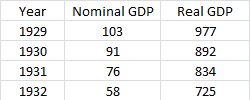# The table below reports nominal and real GDP for the U.S. from 1929 to 1932. What was the growth...

## Question:

The table below reports nominal and real GDP for the U.S. from 1929 to 1932.What was the growth rate of real GDP for 1931?

a. -6.93%, Real GDP is a better gauge of economic well-being than nominal GDP.

b. -6.49%, Nominal GDP is a better gauge of economic well-being than real GDP.

c. -6.93%, Nominal GDP is a better gauge of economic well-being than real GDP.

d. -6.49%, Real GDP is a better gauge of economic well-being than nominal GDP.

## Gross Domestic Product:

The gross domestic product (GDP) is the value of all the goods and services produced within the territory of a country during a certain period. The GDP is normally computed at least once a year.

The correct answer is d. -6.49%, Real GDP is a better gauge of economic well-being than nominal GDP.

Let us determine the growth rate of real GDP for 1931. The formula is:

• Growth rate = (Real GDP in 1931 - Real GDP in 1930) / Real GDP in 1930 x 100
• Growth rate = (834 - 892) / 892 x 100 = - 6.49%

Therefore, the growth rate of the real GDP for 1931 is - 6.49.

In determining the well-being of an economy, the real GDP is a better measurement than nominal GDP. This is because the real GDP considers inflation. Therefore, it tells us the real value of the goods and services.